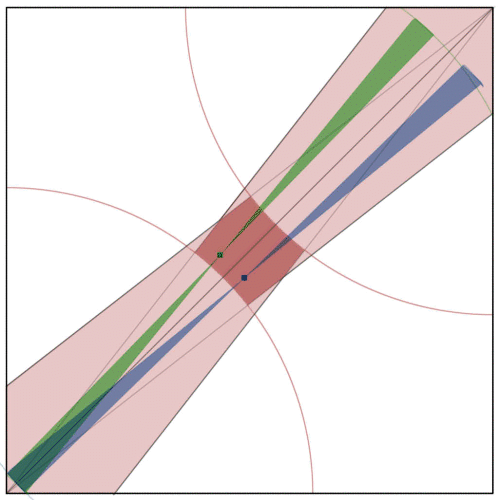BrainDen.com - Brain Teasers

## Question

Inspired by BMAD's Pickup Sticks puzzle, I ask the following related question.

Into a unit square you drop two needles (having negligible width) of length L. (0 < L < sqrt(2)). Consider the probability p( L ) that they will intersect. A moment's thought reveals that the probability approaches zero as L approaches zero.  p( L ) is a strictly increasing function and approaches a limit as approaches sqrt (2).

What is the maximum value of  p( L )?

Clue

Spoiler

This is as much a logic puzzle as a mathematical one.

## Recommended Posts

• 0

purely a guess at the approach but

Reveal hidden contents

Spoiler

When the needles have a length that is close to the length of the diagonal, at least half of the time they must intersect.

##### Share on other sites
• 0
8 hours ago, phaze said:

must the entire needle fit into the unit square?

Reveal hidden contents

Spoiler

There are three cases for the needles: (1) they don't touch, or they touch by (2) intersecting or by (3) overlapping.

When the needles have any "wiggle room" at all, the probability that they will touch by overlapping (which requires *exactly* the same angular orientation) is zero. When they don't have any wiggle room, (when their length is exactly that of the diagonal) there are only two positions available to them. In that case, they always touch: half of the time by intersecting, the other half by overlapping.

The OP states that 0 < L < sqrt(2). Since the interval is open they always have some wiggle room. Thus for lengths near (but less than) the diagonal length, (1) overlap still has probability zero, and (2) intersection is possible in the almost-parallel case (as well as being certain in the almost-perpendicular case.) So the intersection probability for long needles is always greater than 0.5. How much greater can it be?

##### Share on other sites
• 0

0.9999...

If L = sqrt(2), they must touch by overlapping or by being perpendicular.

As it approaches sqrt(2), there becomes less and less room for them to not intersect, and the probability of them not intersecting becomes less and less.  So I guess it stands to reason that the answer will be approaching 1.

Edited by Molly Mae
##### Share on other sites
• 0
19 hours ago, Molly Mae said:

Hide contents

0.9999...

If L = sqrt(2), they must touch by overlapping or by being perpendicular.

As it approaches sqrt(2), there becomes less and less room for them to not intersect, and the probability of them not intersecting becomes less and less.  So I guess it stands to reason that the answer will be approaching 1.

In this puzzle, limits can lead us astray.

Spoiler

Concerning large values of L:

So long as L < sqrt (2), by even the smallest of amounts, a needle will fall into (one of two) narrow regions of non-zero area that contains a diagonal. A needle can lie in that region without intersecting the diagonal. That means a second needle may lie in the same region, but on the opposite side of the diagonal, Two needles in the same region thus need not intersect. But if L = sqrt(2) two needles in the same region must overlap. This makes the intersection probability discontinuous at sqrt(2), Because it is discontinuous, it does not possess a limit there.

##### Share on other sites
• 0
On 4/22/2016 at 11:18 AM, BMAD said:

Hide contents

2/pi

It turns out to rational. When the two sticks lie close to the opposite diagonals they always intersect, when they're near the same diagonal they intersect with a very simple probability that I think can be proved geometrically

##### Share on other sites
• 0

Simulation shows the probability to be 0.75.

This corresponds to 100% intersection if the needles align with opposite diagonals and 50% if the align with the same diagonal.
Here is a figure that suggests how this comes about:We chose here a needle length of about 1.26. A simple construction shows (light red) the area where a needle of that length could land and (dark red) the region that its center point can occupy. The center point of a green needle landed on the green dot. The green bow-tie is the region the needle can occupy with its center fixed at the dot. The blue dot is a reflection in the diagonal, and the blue bow tie is the region the mirror image of the green needle can occupy. Note the coincidence of the lower left boundaries of the bow-ties. As the green needle moves through its allowable positions in a CW motion, the blue needle does the same in a CCW motion. During exactly 1/2 of that motion the two needles intersect. During the other 1/2 they do not. This is only a suggestive proof. The bow-ties can take different locations for different landing points. In some cases the blue and green needles never intersect, in others they always intersect and in still others they intersect for fractions of their motion that differ from 1/2. All the cases average out to 1/2.

## Join the conversation

You can post now and register later. If you have an account, sign in now to post with your account.×   Pasted as rich text.   Paste as plain text instead

Only 75 emoji are allowed.

×   Your previous content has been restored.   Clear editor

×   You cannot paste images directly. Upload or insert images from URL.